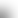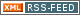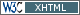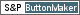www.LinuxHowtos.org howtos, tips&tricks and tutorials for linuxfrom small one page howto to huge articles all in one place

search text in:

Poll
Which kernel version do you use?

poll results

using iotop to find disk usage hogs

using iotop to find disk usage hogs

words:

887

views:

165658

userrating:

average rating: 1.8 (88 votes) (1=very good 6=terrible)

May 25th. 2007:
Words

486

Views

248876

Workaround and fixes for the current Core Dump Handling vulnerability affected kernels

Workaround and fixes for the current Core Dump Handling vulnerability affected kernels

words:

161

views:

133639

userrating:

average rating: 1.3 (28 votes) (1=very good 6=terrible)

April, 26th. 2006:

You are here: manpages

# UNITS

Section: Linux Programmer's Manual (7)
Updated: 2017-09-15

## NAME

units - decimal and binary prefixes

## DESCRIPTION

### Decimal prefixes

The SI system of units uses prefixes that indicate powers of ten. A kilometer is 1000 meter, and a megawatt is 1000000 watt. Below the standard prefixes.
 Prefix Name Value y yocto 10^-24 = 0.000000000000000000000001 z zepto 10^-21 = 0.000000000000000000001 a atto 10^-18 = 0.000000000000000001 f femto 10^-15 = 0.000000000000001 p pico 10^-12 = 0.000000000001 n nano 10^-9 = 0.000000001 mc micro 10^-6 = 0.000001 m milli 10^-3 = 0.001 c centi 10^-2 = 0.01 d deci 10^-1 = 0.1 da deka 10^ 1 = 10 h hecto 10^ 2 = 100 k kilo 10^ 3 = 1000 M mega 10^ 6 = 1000000 G giga 10^ 9 = 1000000000 T tera 10^12 = 1000000000000 P peta 10^15 = 1000000000000000 E exa 10^18 = 1000000000000000000 Z zetta 10^21 = 1000000000000000000000 Y yotta 10^24 = 1000000000000000000000000

The symbol for micro is the Greek letter mu, often written u in an ASCII context where this Greek letter is not available. See also

### Binary prefixes

The binary prefixes resemble the decimal ones, but have an additional aqiaq (and "Ki" starts with a capital aqKaq). The names are formed by taking the first syllable of the names of the decimal prefix with roughly the same size, followed by "bi" for "binary".
 Prefix Name Value Ki kibi 2^10 = 1024 Mi mebi 2^20 = 1048576 Gi gibi 2^30 = 1073741824 Ti tebi 2^40 = 1099511627776 Pi pebi 2^50 = 1125899906842624 Ei exbi 2^60 = 1152921504606846976

### Discussion

Before these binary prefixes were introduced, it was fairly common to use k=1000 and K=1024, just like b=bit, B=byte. Unfortunately, the M is capital already, and cannot be capitalized to indicate binary-ness.

At first that didn't matter too much, since memory modules and disks came in sizes that were powers of two, so everyone knew that in such contexts "kilobyte" and "megabyte" meant 1024 and 1048576 bytes, respectively. What originally was a sloppy use of the prefixes "kilo" and "mega" started to become regarded as the "real true meaning" when computers were involved. But then disk technology changed, and disk sizes became arbitrary numbers. After a period of uncertainty all disk manufacturers settled on the standard, namely k=1000, M=1000 k, G=1000 M.

The situation was messy: in the 14k4 modems, k=1000; in the 1.44 MB diskettes, M=1024000; and so on. In 1998 the IEC approved the standard that defines the binary prefixes given above, enabling people to be precise and unambiguous.

Thus, today, MB = 1000000 B and MiB = 1048576 B.

In the free software world programs are slowly being changed to conform. When the Linux kernel boots and says

hda: 120064896 sectors (61473 MB) w/2048KiB Cache

the MB are megabytes and the KiB are kibibytes.

## Index

NAME
DESCRIPTION
Decimal prefixes
Binary prefixes
Discussion
COLOPHON||- Powered by- Running on-
Copyright 2004-2020 Sascha Nitsch Unternehmensberatung GmbH::- Level Triple-A Conformance to Web Content Accessibility Guidelines 1.0 -
- Copyright and legal notices -